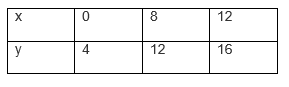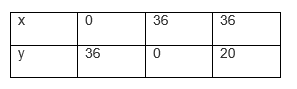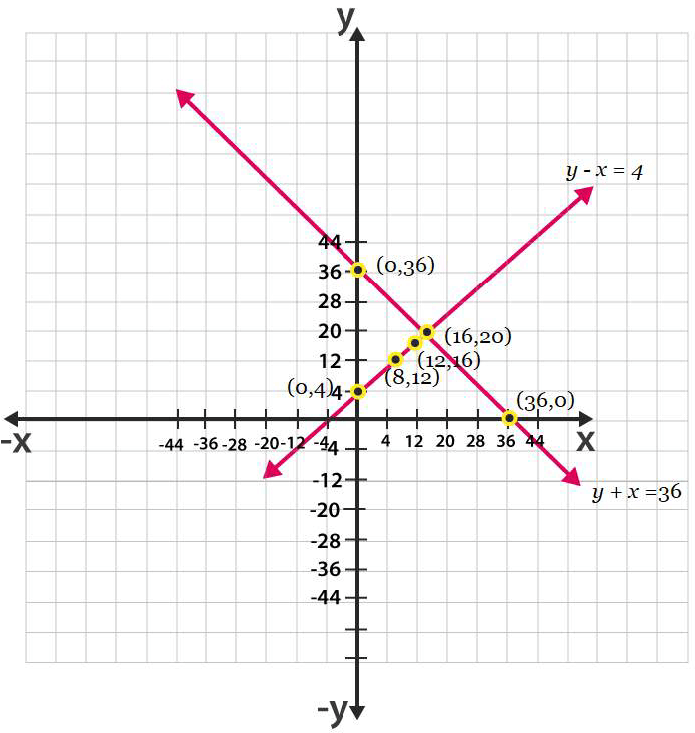Guru

# Half the perimeter of a rectangular garden, whose length is 4 m more than its width, is 36 m. Find the dimensions of the garden. Q.5

• 2

This is very important question of class 10 of exercise 3.2 . How can i solve this question. I want to get the best way of answer for solving this question Half the perimeter of a rectangular garden, whose length is 4 m more than its width, is 36 m. Find the dimensions of the garden.

Share

1. Let us consider.

The width of the garden is x and length is y.

Now, according to the question, we can express the given condition as;

y – x = 4

and

y + x = 36

Now, taking y – x = 4 or y = x + 4For y + x = 36, y = 36 – xThe graphical representation of both the equation is as follows;From the graph you can see, the lines intersects each other at a point(16, 20). Hence, the width of the garden is 16 and length is 20.

• 2
2. This is the video solution for question 5, Exercise 3.2  Pair of linear equations in two variables| NCERT Maths Solutions| Class10

• 0